# Wide-Angle Beam Propagation Method based on Pade Approximant Operators

Compatibility:

Here, we follow the Padé approximant approach to get the wide angle beam propagation .

It is worth it to point out that expansion via Padé is more accurate than Taylor expansion for the same order of terms. When Padé is employed, larger angles, higher index contrast and more complex mode interference can be analyzed in both guided wave and free space as the Padé order increases. See below the guidelines for using the Padé technique:

We may formally rewrite Equation 50 in the form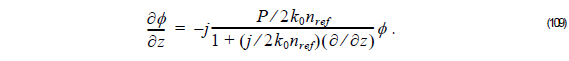Equation 109 can be reduced to the following expression: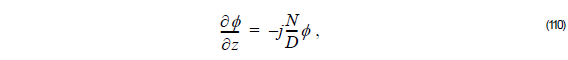where N and  are both polynomials of the operator P.
Differentiating Equation 110 based on FD scheme, we get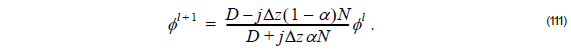The parameter α is introduced to control the finite difference scheme. The range of α is between 0 and 1. We can have standard implicit scheme with α = 0 , explicit scheme with α = 1 and Crank-Nicolson scheme with α = 0.5. For Crank-Nicolson scheme the propagating field does not diverge as the beam propagates.

The numerator of the term on the right-hand side of Equation 111 can be factorized as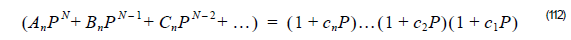where the coefficients c1, c2, …, cn  can be obtained by solving the algebraic equations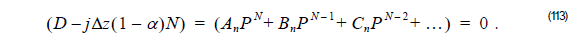The denominator of the term on the right-hand side of Equation 111 can be factorized as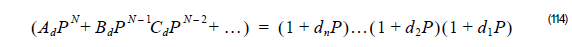where the coefficients d1, d2, …dn can be obtained by solving the algebraic equationHere, c0   = d0   = P0  = 1

Thus, the unknown field φ l + 1  at z + Δz is related to the known field φ l at z as followsorIn general, determination of the c ‘s and d ‘s requires the one-time solution of an n th-
order complex algebraic equation.

The explicit expressions for the corresponding Wide-angle (WA) orders using Padé approximant operator are shown below.
The term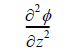in Equation 109 can be taken into account quite effectively by using the recursion relation:If Equation 118 is now used to replace the z derivative in the denominator of Equation 118 we can get the following explicit expressions for several wide-angle (WA) orders.